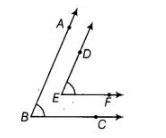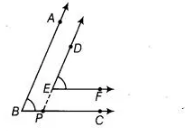# In the figure, BA || ED and BC || EF.`
Question:

In the figure, BA || ED and BC || EF. Show that ∠ABC = ∠DEF.Solution:

Given BA || ED and BC || EF.

To show ∠ABC = ∠DEF.

Construction Draw a ray EP opposite to ray ED.Proof In the figure, $B A \| E D$ or $B A \| D P$

$\therefore \quad \angle A B P=\angle E P C$ [corresponding angles]

$\Rightarrow \quad \angle A B C=\angle E P C$ $\ldots($ i)

Again, $B C \| E F$ or $P C \| E F$

$\therefore \quad \angle D E F=\angle E P C \quad$ [corresponding angles] ...(ii)

From Eqs. (i) and (ii),

$\angle A B C=\angle D E F$